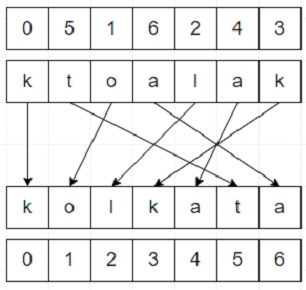# Program to shuffle string with given indices in Python

Suppose we have a string s and a list of indices ind, they are of same length. The string s will be shuffled such that the character at the position i, moves to indices[i] in the final string. We have to find the final string.

So, if the input is like s = "ktoalak" ind = [0,5,1,6,2,4,3], then the output will be "kolkata"To solve this, we will follow these steps −

• fin_str := a list whose size is same as s and fill with 0

• for each index i and character v in s, do

• fin_str[ind[i]] := v

• join each character present in fin_str and return

## Example (Python)

Let us see the following implementation to get better understanding −

Live Demo

def solve(s, ind):
fin_str =  * len(s)
for i, v in enumerate(s):
fin_str[ind[i]] = v

return "".join(fin_str)

s = "ktoalak"
ind = [0,5,1,6,2,4,3]
print(solve(s, ind))

## Input

"ktoalak", [0,5,1,7,2,4,3]

## Output

kolkata